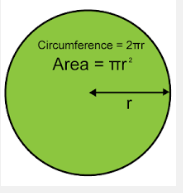Geometry Review For Grade 8: Circles + Angle Measurement

10 Questions | Total Attempts: 250SettingsGeometry to me is among the simplest subjects in Mathematics and this is because it is more practical and uses tools. How about you? Complete this online review assessment to below and find out how good you are at reviewing the facts of grade 7 Geometry.

• 1.
Name the Angle
• A.

HGF OR FGH

• B.

HFG OR GFH

• C.

HFG OR GFH

• 2.
Find the Area of the Circle. Round to the nearest tenth.
• A.

A= 157 squared cm

• B.

A= 31.4 squared cm

• C.

A= 15.7 squared cm

• D.

A = 78.5 squared cm

• 3.
Name the Angle
• A.

TVU ORUVT

• B.

VUT OR TUV

• C.

VTU OR UTV

• 4.
True or False. The radius of the circle is 4m
• A.

True

• B.

False

• 5.
Find the Circumference of the Circle. Round to the nearest tenth.
• A.

C= 75.36 ft

• B.

C=75. 4 ft

• C.

C= 37.7 ft

• D.

C= 452.2 ft

• 6.
FIND THE MEASURE OF ANGLE XUSE A PROTRACTOR TO MEASURE THE ANGLE IF NEEDED
• A.

X = 100 degrees

• B.

X = 105 degrees

• C.

X = 15 degrees

• D.

X = 25 degrees

• E.

ANGLE X IS BETWEEN 120 AND 130 DEGREES

• F.

X = 285 degrees

• 7.
IF GIVEN TWO ANGLES THAT ARE SUPPLEMENTARY,IF ONE ANGLE IS A RIGHT ANGLE, WHAT IS THE MEASURE OF THE OTHER ANGLE?
• A.

270 DEGREES

• B.

90 DEGREES

• C.

45 DEGREEES

• D.

60 DEGREES

• 8.
If two angles are complementary and one of them is 77°, what is the size of the other angle?
• A.

57 DEGREES

• B.

167 DEGREES

• C.

13 DEGREES

• D.

103 DEGREES

Related TopicsBack to top